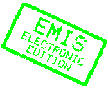MATHEMATICA BOHEMICA, Vol. 130, No. 2, pp. 113-134 (2005)

# On the Boolean function graph of a graph and on its complement

## T. N. Janakiraman, S. Muthammai, M. Bhanumathi

T. N. Janakiraman, National Institute of Technology, Tiruchirappalli, 620 015, India, e-mail: janaki@nitt.edu; S. Muthammai, M. Bhanumathi, Government Arts College for Women, Pudukkottai, 622 001, IndiaAbstract: For any graph \$G\$, let \$V(G)\$ and \$E(G)\$ denote the vertex set and the edge set of \$G\$ respectively. The Boolean function graph \$B(G,L(G),\NINC)\$ of \$G\$ is a graph with vertex set \$V(G)\cup E(G)\$ and two vertices in \$B(G,L(G),\NINC)\$ are adjacent if and only if they correspond to two adjacent vertices of \$G\$, two adjacent edges of \$G\$ or to a vertex and an edge not incident to it in \$G\$. For brevity, this graph is denoted by \$B_1(G)\$. In this paper, structural properties of \$B_1(G)\$ and its complement including traversability and eccentricity properties are studied. In addition, solutions for Boolean function graphs that are total graphs, quasi-total graphs and middle graphs are obtained.

Keywords: eccentricity, self-centered graph, middle graph, Boolean function graph

Classification (MSC2000): 05C15

Full text of the article:

[Next Article] [Contents of this Number] [Journals Homepage]
© 2005–2010 FIZ Karlsruhe / Zentralblatt MATH for the EMIS Electronic Edition